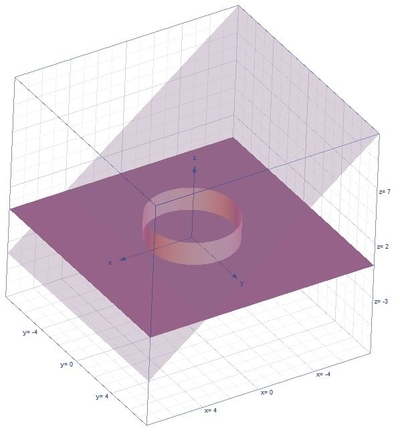# Determine the volume of the solid bounded inside {eq}\displaystyle x^2 + y^2 = 9, {/eq} above {eq}\displaystyle z = 0 {/eq} and below {eq}\displaystyle x + z = 4 {/eq}

## Question:

Determine the volume of the solid bounded inside {eq}\displaystyle x^2 + y^2 = 9, {/eq} above {eq}\displaystyle z = 0 {/eq} and below {eq}\displaystyle x + z = 4 {/eq}

## Volume of the Region:

Let us find the volume of the region using the triple integrals formula in cylindrical coordinates which is {eq}V=\int_{\alpha }^{\beta }\int_{h_{1}(\theta )}^{h_{2}(\theta )}\int_{u_{1}(r\cos \theta ,r\sin \theta ,z)}^{u_{2}(r\cos \theta ,r\sin \theta ,z)}r\:f\left ( r\cos \theta ,r\sin \theta ,z \right )dzdrd\theta {/eq}

and the conversion formulas are the following {eq}r^{2}=x^{2}+y^{2},\:x=r\cos \theta ,\:y=r\sin \theta {/eq}.

Become a Study.com member to unlock this answer!

Below is the graph,From the given equations the limits are the following,

{eq}0\leq \theta \leq 2\pi ,\:0\leq r\leq 3,\:0\leq z\leq...